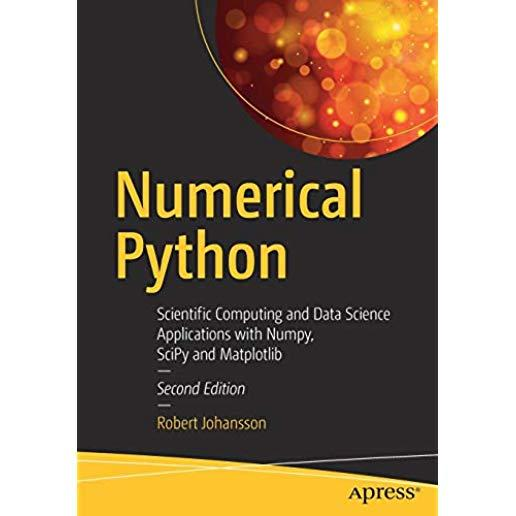### Numerical Python: Scientific Computing and Data Science Applications with Numpy, Scipy and Matplotlib### Huge savings for students

Each student receives a 50% discount off of most books in the HSG Book Store. During class, please ask the instructor about purchase details.
 List Price: \$54.99 Price: \$27.50 You Save: \$27.50
9

Leverage the numerical and mathematical modules in Python and its standard library as well as popular open source numerical Python packages like NumPy, SciPy, FiPy, matplotlib and more. This fully revised edition, updated with the latest details of each package and changes to Jupyter projects, demonstrates how to numerically compute solutions and mathematically model applications in big data, cloud computing, financial engineering, business management and more.

Numerical Python, Second Edition, presents many brand-new case study examples of applications in data science and statistics using Python, along with extensions to many previous examples. Each of these demonstrates the power of Python for rapid development and exploratory computing due to its simple and high-level syntax and multiple options for data analysis.

After reading this book, readers will be familiar with many computing techniques including array-based and symbolic computing, visualization and numerical file I/O, equation solving, optimization, interpolation and integration, and domain-specific computational problems, such as differential equation solving, data analysis, statistical modeling and machine learning.

What You'll Learn

• Work with vectors and matrices using NumPy
• Plot and visualize data with Matplotlib
• Perform data analysis tasks with Pandas and SciPy
• Review statistical modeling and machine learning with statsmodels and scikit-learn
• Optimize Python code using Numba and Cython
Who This Book Is For

Developers who want to understand how to use Python and its related ecosystem for numerical computing.

Apress